# Area Of Hemisphere

A hemisphere is obtained when we cut the sphere into two equal halves. The total surface area of hemisphere will be equal to the sum of its curved surface area and the base area of hemisphere. The base is in circular shape here. We can find various real-life examples of the hemispheres such as our planet Earth can be divided into two segments the southern & northern hemispheres.

 Surface Area of Hemisphere = 3 π r2

The Hemispheres can either be of a solid type or can be hollow.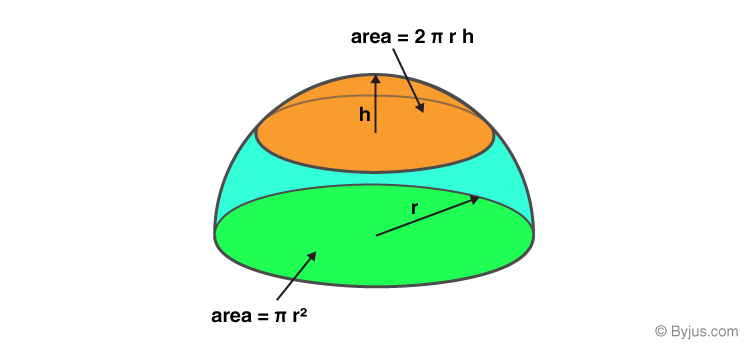## Surface Area of Hemisphere

The surface area is the area occupied by the surface of the solid object. The surface area is classified into two types, namely;

• Curved Surface Area (CSA)
• Total Surface Area (TSA)

### Curved Surface Area

As the Hemisphere is the half part of a sphere, therefore, the curved surface area is also half that of the sphere.

Curved surface area of hemisphere =  1/2 ( 4 π r2) = 2 π r2

### Base Area

The base of the hemisphere is in circular shape. As we know by the formula of area of circle,

Area of base = π r2

### Total Surface Area

The total surface area of the hemisphere– While calculating the total surface area of a hemisphere, we need to consider the base of the hemisphere which is circular. Thus, the total surface area of a hemisphere is equal to:

Total Surface Area (TSA) = Curved Surface Area + Area of the Base Circle

= 2 π r2 + π r2

= 3 π r2

### Area of Hollow Hemisphere

A hollow hemisphere has two diameters for its circular bases, one is for inside circular base (hollow part) and one is for outside circular base (rigid part). Therefore, the area of hollow hemisphere is equal to the difference between area of external hemisphere and area of internal hemisphere.

Area of Hollow Hemisphere = Area of External Hemisphere – Area of Internal Hemisphere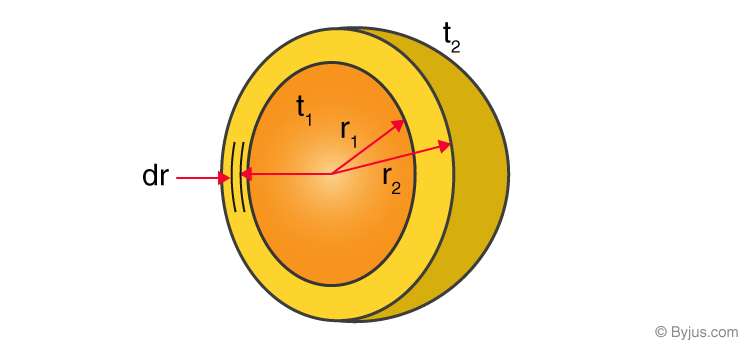From the above figure, we can see, r1 is the radius of internal hemisphere and r2 is the radius of external hemisphere.

We can derive the formula by adding the curved surfaces of outer hemisphere, inner sphere and the ring formed between them.

Curved Surface of outer hemisphere = 2π r22

Curved Surface of inner hemisphere = 2π r12

Area of the ring = π(r22 – r12)

Therefore, total area of hollow hemisphere is:

TSA = 2π r2+ 2π r1+ π(r22 – r12)

TSA = 2π (r2+ r12) + π(r22 – r12)

### Real-Life Examples of Hemisphere

In the pictures given below, bowl and coconut shells are examples of hollow-hemisphere.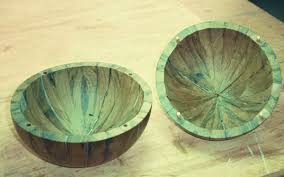Coconut Shell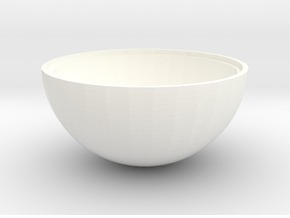Bowl

This is all about the area formulae of the Hemispheres. To know more about the other geometrical shapes and to find Surface Areas and Volume, visit our site BYJU’S.

## Video Lesson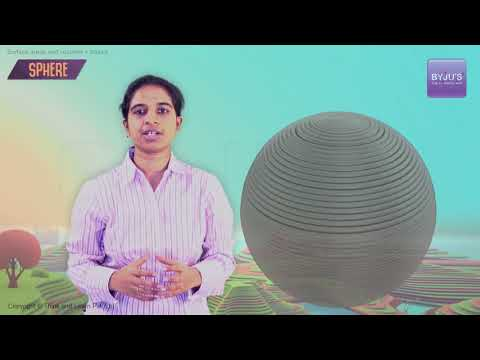## Solved Examples on Area of Hemisphere

Example 1:

Find the total surface area of the hemisphere whose base radius is 3 cm (take 𝜋 = 3.14).

Solution:

Base radius of the hemisphere = 3 cm

The total surface area of a hemisphere = 3𝜋r2

= 3 × 3.14 × 32

= 84.78 cm2.

Example 2:

Find the total surface area of a hollow hemisphere whose inner and outer radii are 7 cm and 8 cm, respectively.

Solution:

Let the inner and outer radii of the hemisphere be r1 and r2, respectively.

Then r1 = 7 cm and r2 = 8 cm

The total surface area of a hollow cylinder = 2𝜋(r22 + r12) + 𝜋(r22 – r12

= 2𝜋 (82 + 72) + 𝜋(82 – 72)

= 2𝜋 (64 + 49) + 𝜋(64 – 49)

= 2𝜋 × 113 + 15𝜋

= 241𝜋 cm2.

Example 3:

Find the base area of a cylinder whose curved surface area is 256 cm2.

Solution:

Let r be the radius of the hemisphere.

The curved surface area of a hemisphere = 2𝜋r2 = 256 cm2

Base radius of the hemisphere = 𝜋r2 = CSA of hemisphere/2 = 256/2 = 128 cm2.

## Practice Questions on Area of Hemisphere

1. Find the total surface area of a solid hemisphere whose volume is 19,404 cm3
2. Find the surface area of a hollow hemisphere whose inner and outer diameter is 12 cm and 14 cm, respectively.
3. Find the capacity of a hemispherical bowl whose curved surface area is 344 cm2.

## Frequently Asked Questions on Area of Hemisphere

Q1

### What is the area and volume of a hemisphere?

The area of a hemisphere is of two types:
The curved surface area of a hemisphere = 2𝜋r2 square units.
The total surface area of a hemisphere = 3𝜋r2 square units.
The volume of a hemisphere is determined by the formula (⅔)𝜋r3 cubic units.

Q2

### What is the total surface area of a hollow hemisphere?

The total surface area of a hollow hemisphere whose inner and outer radii are r1 and r2, respectively, is given by 2𝜋(r22 + r12) + 𝜋(r22 – r12) square units.

Q3

### What is the formula for the base radius of a hemisphere?

The formula for the base radius of a hemisphere is 𝜋r2, where r is the radius of the hemisphere.

Q4

### What is the difference between the curved surface area and the total surface of a hemisphere?

The curved surface area is the surface area of only the curved part of the hemisphere whereas the total surface area is the sum of the curved surface and the base radius of the hemisphere.

Q5

### What is the lateral surface area of a hemisphere?

The lateral surface area is also known as the curved surface area of the hemisphere. The curved surface area of the hemisphere is 2𝜋r2 where r is the radius of the hemisphere.

Test your Knowledge on Area of Hemisphere